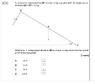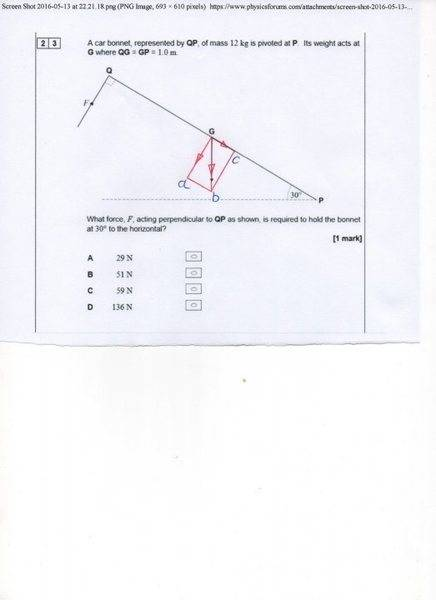# Moment Question / inclined triangle

• Sabeshan Ratneswaran

## Homework Statement

In this I tried to resolve the components.

So first thing, I converted the 12kg into Newtons so it would be 117.72 Newtons. Then found the perpendicular distance which is to g: cos(30)x1 then multiply the answer by 117.2N to give the weight down as 102N. As the moment principle state that clockwise have to be equal to anticlockwise the vertical of the Q have to 102N then i found the tension by 102/cos(30) which will give the final answer as 118N. But this answer is not one of the choices so it is wrong.

Can someone explain to me where I have gone wrong.

Thank You.

#### Attachments

•Screen Shot 2016-05-13 at 22.21.18.png
15.4 KB · Views: 588

o give the weight down as 102N.
No, the weight down is mg. In what direction is there a force mg cos(30)?
To balance the moments, pick an axis. Which axis did you use?

So first thing, I converted the 12kg into Newtons so it would be 117.72 Newtons.
You found the weight of the 12kg mass. Good.

Then found the perpendicular distance which is to g: cos(30)x1 then multiply the answer by 117.2N to give the weight down as 102N.
You found the anticlockwise torque (moment) due to the weight of that bonnet. Good. (Not the "weight" down, the moment.)

As the moment principle state that clockwise have to be equal to anticlockwise
Good.

then i found the tension by 102/cos(30)
Not sure what you did here. What you need to do is find an expression for the clockwise moment due to the force F. (What's the definition of moment?)

You found the weight of the 12kg mass. Good.

You found the anticlockwise torque (moment) due to the weight of that bonnet. Good. (Not the "weight" down, the moment.)

Good.

Not sure what you did here. What you need to do is find an expression for the clockwise moment due to the force F. (What's the definition of moment?)
Hi there,

This is where I am confused. Moment about a pivot is force multiplied by the perpendicular distance between the the pivot and the line of action

We know the vertical component as 102 N so we can resolve to find the tension. This is where I got the expression as 102/cos(30)

We know the vertical component as 102 N so we can resolve to find the tension. This is where I got the expression as 102/cos(30)
No, the vertical force is mg, 117.72N.
You can either take the component of that which is perpendicular to the car bonnet (that will be 102N) and multiply by the distance from the pivot to that force; or take the whole of mg and multiply by the perpendicular (i.e. horizontal) distance from the pivot to the line of action of mg.
You will get the same answer either way. Choose one.

•Sabeshan Ratneswaran
No, the vertical force is mg, 117.72N.
You can either take the component of that which is perpendicular to the car bonnet (that will be 102N) and multiply by the distance from the pivot to that force; or take the whole of mg and multiply by the perpendicular (i.e. horizontal) distance from the pivot to the line of action of mg.
You will get the same answer either way. Choose one.

Quite confused:

is this what you mean 12x9.81xcos(30)x1=102N
Then divide it by cos(30)x2 to give 58.86.

but we are trying to find out the force of F which holds the bonnet equilibrium. By the way the answer is not 59N.

Then divide it by cos(30)x2 to give 58.86
Explain why you divide by that.

•Sabeshan Ratneswaran
Explain why you divide by that.
The principle of moment is the sum of clockwise moment is equal to sum of anticlockwise moment in an equilibrium position. the expression will be FxD=FxD
So basically we are dealing with perpendicular distance so the expression with be [Cos(30)x1] x [9.81x12]=[Cos(30)x2] x F
So making the F the subject will give us 58.86N.

Explain why you divide by that.

I am only dealing with resolved distance components

The principle of moment is the sum of clockwise moment is equal to sum of anticlockwise moment in an equilibrium position. the expression will be FxD=FxD
So basically we are dealing with perpendicular distance so the expression with be [Cos(30)x1] x [9.81x12]=[Cos(30)x2] x F
So making the F the subject will give us 58.86N.
Yes, I understand that, but how do you come to 2 cos(30)? What is the perpendicular distance from the line of action of F to the pivot point?

Yes, I understand that, but how do you come to 2 cos(30)? What is the perpendicular distance from the line of action of F to the pivot point?
It is not from P to F, the distant from P to Q is 2 so resolved it do 2cos(30)..

then I was going to find the value of F by doing 58.86/ cos(30). But i know I have done something wrong.

It is not from P to F, the distant from P to Q is 2 so resolved it do 2cos(30)..
I'll be more specific. Why the cos(30)?

## Homework Statement

In this I tried to resolve the components.

So first thing, I converted the 12kg into Newtons so it would be 117.72 Newtons. Then found the perpendicular distance which is to g: cos(30)x1 then multiply the answer by 117.2N to give the weight down as 102N. As the moment principle state that clockwise have to be equal to anticlockwise the vertical of the Q have to 102N then i found the tension by 102/cos(30) which will give the final answer as 118N. But this answer is not one of the choices so it is wrong.

Can someone explain to me where I have gone wrong.

Thank You.
I'll be more specific. Why the cos(30)?

#### Attachments

Realize that the applied force F is already perpendicular to the distance PQ, so there is no need to take any components.

•Sabeshan Ratneswaran
Realize that the applied force F is already perpendicular to the distance PQ, so there is no need to take any components.
Make sense mentor

Make sense mentor
Look at the diagram. At what angle does F act? What is the perpendicular distance from the pivot point to the line of action of F?

•Sabeshan Ratneswaran
F1 * d1 = F2 * d2 ?Last edited:
•Sabeshan Ratneswaran
Look at the diagram. At what angle does F act? What is the perpendicular distance from the pivot point to the line of action of F?
Thank you for the consistent help.